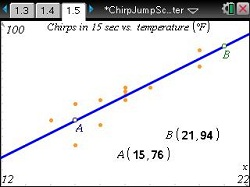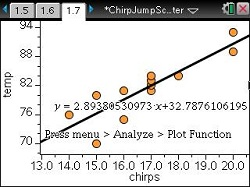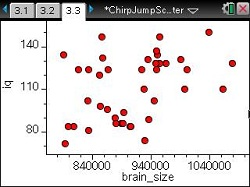# Activities

••• ##### Subject Area

• Math: Algebra I: Data Analysis
• Math: Algebra I: Linear Functions

• ##### Author9-12

15 Minutes

• ##### Device
•TI-Nspire™ CX/CX II
•TI-Nspire™ CX CAS/CX II CAS
• TI-Nspire™
• TI-Nspire™ CAS
• ##### Software

TI-Nspire™
TI-Nspire™ CAS

3.0

## Chirp, Jump, Scatter#### Activity Overview

In this activity, students will find a best fit line for data graphed as scatter plots. Applications of linear relationships provide motivation for students and improve their skills and understanding of finding the equation of a line from two known points. Movable lines make this activity approachable for Algebra 1 students. Students can put into practice what they have learned with extension/homework questions.

#### Key Steps

•In the first problem students will move two points A and B to find a line of best fit on a Graphs & Geometry page. They will use the coordinates to calculate the slope and the equation of the line. Using the equations, students will predict the number of chirps for a given temperature.

•If desired, students can compare their line of best fit with the handheld’s linear regression line by adding both lines to a Data & Statistics page.

•Students will also look at data sets for the Olympic High Jump and Brian Size vs. IQ. They will determine if they can see a trend just by looking at the data or if there is a trend to the data at all. Each problem is followed with applicable question to get students thinking about the data given.